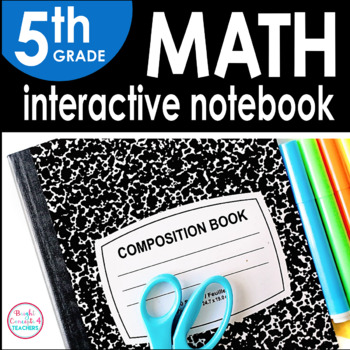# 5th Grade Math Interactive Notebook {Common Core Aligned}5th, Homeschool
Subjects
Standards
Resource Type
Formats Included
• PDF
Pages
142 pages

### Description

Interactive notebooks are a great learning tool in the classroom. They serve as a resource for reviewing standards, a reference tool, or even as an assessment.

This notebook covers all the 5th Grade Common Core MATH standards (including Operations & Algebraic Thinking, Numbers and Operations in Base 10, Geometry and Measurement and Data, Number and Operations-Fractions)

This product includes:

-Notebook cover

-Divider tabs

-Strand Dividers

-Pictures of EVERY page so you can have an example of how each activity can be used

-Student friendly "I can" statements at the top of each page.

I know your students will love making these notebooks as a method to reinforce all the CCSS math standards they have been taught.

Skills covered:

Operations and Algebraic Thinking:

-Order of Operations

-Problem Solving Using Order of Operations

-Writing Numerical Expressions

-Problem Solving Using Numerical Expressions

-Numerical Patterns

-Problem Solving with Numerical Patterns

Numbers and Operations in Base 10:

-Place Value Through Millions

-Problem Solving Using Place Value Through Millions

-Prime Factorization

-Problem Solving Using Prime Factorization

-Powers & Exponents

-Multiplication Patterns

-Multiplying Decimals by Powers of 10

-Problem Solving Using Decimals by Power of 10

-Fractions & Decimals

-Place Value Through Thousandths

-Comparing Decimals

-Ordering Decimals

-Problem Solving Using Decimals

-Rounding Decimals

-Estimating Sums & Differences

-Estimating Products

-Estimating Quotients

-Problem Solving Using Estimating

-Multiplication Properties

-Two-Digit Dividends with Remainders

-Divide Three & Four Digit Dividends

-Quotients with Zero

-Divide Greater Numbers

-Problem Solving Using Remainders

-Problem Solving with Greater Numbers

Geometry:

-Ordered Pairs

-Graph Patterns

-Polygons

-Classifying Triangles

Measurement and Data:

-Converting Units of Length

-Converting Units of Weight

-Converting Units of Capacity

-Problem Solving with Converting Units of Length, Weight, Capacity

-Measurement Data on a Line Plot

-Volume with Unit Cubes

-Volume with Prisms

-Volume of Composite Figures

Numbers and Operations-Fractions:

-Problem Solving Using Addition & Subtraction of Fractions

-Fractions and Division

-Fractions and Bar Diagrams

-Multiplying Whole Numbers and Fractions

-Area of a Rectangle Using Fractions

-Fractions in Simplest Form

-Comparing Fractions

-Fractions and Decimals

-Problem Solving Using Multiplication as Scaling

-Multiplying Mixed Numbers

-Problem Solving Using Multiplication of Fractions & Mixed Numbers

-Dividing Whole Numbers by Unit Fractions

-Problem Solving Using Division of Fractions

More Math Interactive Notebooks:

KINDERGARTEN Math Interactive Notebook

***************************************************

If you would like to get updates on NEW & CURRENT resources...

**************************************************

Email me at brightconcepts4teachers@gmail.com if you have any questions!

Total Pages
142 pages
N/A
Teaching Duration
1 Year
Report this Resource to TpT
Reported resources will be reviewed by our team. Report this resource to let us know if this resource violates TpT’s content guidelines.

### Standards

to see state-specific standards (only available in the US).
Use parentheses, brackets, or braces in numerical expressions, and evaluate expressions with these symbols.
Write simple expressions that record calculations with numbers, and interpret numerical expressions without evaluating them. For example, express the calculation “add 8 and 7, then multiply by 2” as 2 × (8 + 7). Recognize that 3 × (18932 + 921) is three times as large as 18932 + 921, without having to calculate the indicated sum or product.
Generate two numerical patterns using two given rules. Identify apparent relationships between corresponding terms. Form ordered pairs consisting of corresponding terms from the two patterns, and graph the ordered pairs on a coordinate plane. For example, given the rule “Add 3” and the starting number 0, and given the rule “Add 6” and the starting number 0, generate terms in the resulting sequences, and observe that the terms in one sequence are twice the corresponding terms in the other sequence. Explain informally why this is so.
Add and subtract fractions with unlike denominators (including mixed numbers) by replacing given fractions with equivalent fractions in such a way as to produce an equivalent sum or difference of fractions with like denominators. For example, 2/3 + 5/4 = 8/12 + 15/12 = 23/12. (In general, 𝘢/𝘣 + 𝘤/𝘥 = (𝘢𝘥 + 𝘣𝘤)/𝘣𝘥.)
Solve word problems involving addition and subtraction of fractions referring to the same whole, including cases of unlike denominators, e.g., by using visual fraction models or equations to represent the problem. Use benchmark fractions and number sense of fractions to estimate mentally and assess the reasonableness of answers. For example, recognize an incorrect result 2/5 + 1/2 = 3/7, by observing that 3/7 < 1/2.